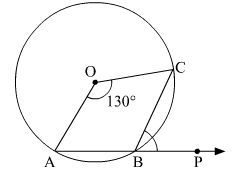# In the given figure, O is the centre of the circle and arc ABC subtends an angle of 130° at the centre.

Question:

In the given figure, O is the centre of the circle and arc ABC subtends an angle of 130° at the centre. If AB is extended to P, find ∠PBC.Solution:

Reflex ∠AOC + ∠AOC = 360
⇒ Reflex ∠AOC + 130 + x = 360
⇒ Reflex ∠AOC = 360 − 130
⇒ Reflex ∠AOC = 230

We know that the angle subtended by an arc of a circle at the centre is double the angle subtended by it on the remaining part of the circle.

Here, arc AC subtends reflex ∠AOC at the centre and ∠ABC at B on the circle.

∴ ∠AOC = 2∠ABC

$\Rightarrow \angle A B C=\frac{230^{\circ}}{2}=115^{\circ}$        ...(1)

Since ABP is a straight line, ∠ABC + PBC = 180
⇒ ∠PBC = 180 − 115
⇒ ∠PBC = 65            ...(2)

Hence, ∠PBC = 65.